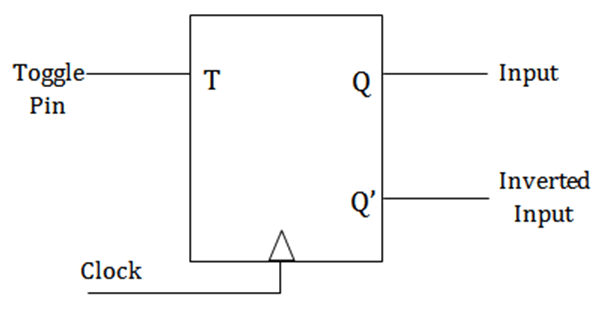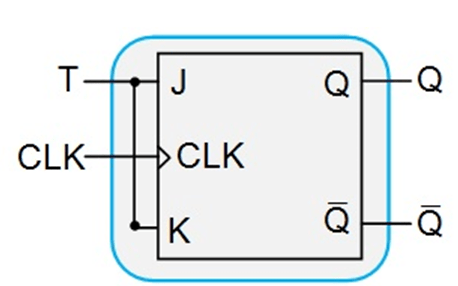# T Flip Flop Explained in Detail

Flip – flops are one of the most fundamental electronic components. These are used as one-bit storage elements, clock dividers and also we can make counters, shift registers and storing registers by connecting the flip flops in particular sequences. These flip flops use feedback concept to create sequential logic where the previous state affect future states (unlike combinational circuit).

An essential characteristic of flip flops is that it changes its state whenever there is a positive or negative transition on the control signal. Also, we need to define the input-output relationship here. The common types of flip flops are as follows:

Among the above four, only D and J-K flip flops are available in the integrated IC form and have immense applications.

## T Flip Flop

The name of this flip flop came from its toggling nature. Most applications of T flip flops are in counters and control circuits. The disadvantage of J-K flip flop gave rise to the concept of J-K flip flop. Thus, J-K flip flop with certain modifications to make it work in the toggling region can be called as T flip flop.symbol of T Flip-flop

Anytime when the clock signal is low, the input never affects the output state. So when the clock is high then only input gets active. Here clock signal is the control signal and thus T flip flop is a bi-stable latch. It has two stable output states based on the inputs which have been discussed below.

### Truth table of T Flip Flop

 Input Output Present State Next State T Qn Qn+1 0 0 0 0 1 1 1 0 1 1 1 0

As told above, J-K flip flop with certain modifications is T flip flop. Q and Q’ are the output states of the flip flop. We can see from the table that with the change in input, output state changes. But point to note here is all these happen in the presence of the clock signal. For complementary input, its working is different from that of J-K & S-R flip flops. This only has a toggling function.

We can modify the input-output relationship of a flip flop by adding logic gates and appropriate interconnections.

In simple words, T flip flop operates as:

When input T is low, both present and next state will be same:

• T = 0, present state = 0 & next state = 0
• T = 1, present state = 1 & next state = 1

When input T is high, next state will be the inverse of the present state:

• T = 0, present state = 0 & next state = 1
• T = 1, present state = 1 & next state = 0

## From SR or JK to T Flip Flop

If we have an SR flip flop, then by using two AND gates inappropriate places we can achieve the required T flip flop.Conversion of S R flip flop to T flip flop

The process for JK flip flop is even easier. No additional gates are required and we just need to connect the same input signal to both input pins.Conversion of J K flip flop to T flip flop

### From D to T Flip Flop

For converting D flip flop to T flip flop, connect an XOR gate between T and Q and give its output as input to D.Conversion of D flip flop to T flip flop

Let us now see 2 important applications of T flip flop.

• 2 – bit parallel load registers
• Frequency Division Circuit

### 2- bit Parallel Load Register

For storing data, we generally prefer shift registers & registers. But, the primary concern is always about the size. Therefore, this 2 – bit parallel load register instead of the 4 -bit register can be a help.

So, we mainly have two tasks here: holding the data & loading it in parallel. I guess you should be able to tell that how to hold the data.

Yes, it is easy if we keep the input T = 0.

Now comes the difficult part of loading it in parallel. The value of X should go to the output of the flip flop. We need to use a 2 x 1 MUX here. We XOR the present state output with the X input and give it to the MUX. The other input for MUX will be a logic low signal (a constant 0). Finally, the output of the MUX is connected to the input of the T flip flop. 2 such combinations are needed as it is a 2 bit register.

### Frequency Division Circuit

The T flip flops are useful when we need to reduce the frequency of the clock signal. If we use the original clock as flip flop clock and keep the T input at logic high then the output changes state once per clock period. This is with the assumption that the flip flop is not sensitive to both clock edges. This makes the output clock frequency as half of the frequency of the input clock. So the T flip flop works as a “Frequency Divider Circuit”.

Now, a question should arise in your mind that how to make the circuit for the frequency divider!

Isn’t it?

So the answer is by giving the Q’ as feedback to the input T.

Ajay Dheeraj

(Technical Content Developer)

•
•
•
•
•
•